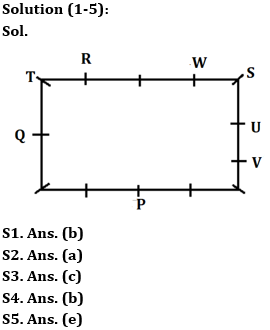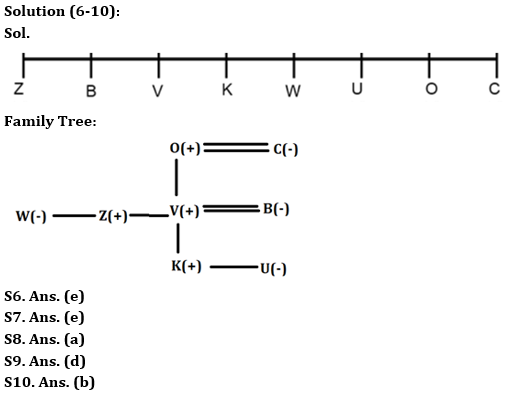Latest Banking jobs   »

# Reasoning Ability Quiz For LIC ADO Mains 2023-30th March

Directions (1-5): Answer the questions based on the information given below.
Certain numbers of persons are sitting around the rectangular table facing towards the center. At least 1 person sit on each side and at most 3 persons sit on each side. One person sits at each corner.
U sits fourth to the right of P who sits on the longer side. T sits opposite to one, who sits at the corner of the table. R sits second to the right of W such that both sit on the same side. One person sits between U and W. Q sits immediate right of T. No one sits on the same side as of Q. Two persons sit between Q and P. V sits immediate left of U. S sits second to the right of V. Only one person adjacent to V sits on the side.

Q1. How many persons sit around the table?
(a) 20
(b) 13
(c) 12
(d) 10
(e) 9

Q2. Who sits immediate left of T?
(a) R
(b) W
(c) U
(d) V
(e) P

Q3. How many persons sit between R and V when counted from the left of R?
(a) Six
(b) Three
(c) Four
(d) One
(e) Five

Q4. Who sits third to the left of P?
(a) S
(b) Q
(c) T
(d) R
(e) W

Q5. How many persons sit between W and R when counted from the left of W?
(a) Six
(b) Five
(c) Four
(d) Eight
(e) Ten

Direction (6-10): Study the following information and answer the given questions.
There are eight members in a family – V, W, O, B, U, C, K, and Z. Each of them has a relationship with V – mother, sister, wife, son, father, brother and daughter but not necessarily in the same order. They are sitting in a single line facing North.
C is an immediate neighbour of V’s father. Two people are sitting between U and W’s brother. Two people sit between V’s son and V’s father. C is sitting at the right end of the line. Z’s father sits to the immediate right of V’s daughter. V is not an immediate neighbour of U. V’s wife is an immediate neighbour of Z. K is younger than O. V’s mother sits at one of the ends of the line. U sits second to the right of V’s son. B and V’s brother are sitting together. V’s brother sits second to the left of V. Two people are sitting between B and V’s sister.

Q6. How many people are sitting between U’s mother and V?
(a) One
(b) Two
(c) Four
(d) Three
(e) None

Q7. Who is V’s brother’s sister?
(a) O
(b) C
(c) Z
(d) U
(e) W

Q8. Who is V’s wife?
(a) B
(b) W
(c) C
(d) U
(e) Z

Q9. Who sits second to right of V’s sister?
(a) V
(b) C
(c) U
(d) O
(e) None of these

Q10. How many people are sitting between B and V’s daughter?
(a) Two
(b) Three
(c) Five
(d) Six
(e) One

Direction (11-13): In the following questions assuming the given statement to be true, find which of the conclusion(s) among given conclusions is/are definitely true and then give your answers accordingly.

Q11. Statements: F = A > B; C ≥ D; G < E ≤ D; C ≤ B; G=R >H
Conclusions: I. C ≥ R
II. A > G
III. F ≥ D
(a) Only I is true
(b) Only II is true
(c) Either I or III is true
(d) None is true
(e) Both II and III are true

Q12. Statements: R > C ≥M = N < T ≤ S; A ≥ B =D < N = J ≥P
Conclusions: I. P < S
II. A > T
III. R ≥ D
(a) Only I is true
(b) Only III is true
(c) Either I or II is true
(d) Both II and III are true
(e) Both I and II are true

Q13. Statements: O > M = P ≤ W < K; P > F ≥ U; S <U
Conclusions: I. O > U
II. M > K
III. S ≤ W
(a) Only I is true
(b) Only II is true
(c) Either II or III is true
(d) Only III is true
(e) Both I and II are true

Directions: (14-15): Answer the questions based on the information given below.
A % B means A is not smaller than B
A@B means A is neither smaller than nor equal to B
A & B means A is neither greater than nor equal to B
A\$B means A is not greater than B
A# B means A is neither smaller than nor greater than B

Q14. Statements: A%B#R@Q&S\$N, N%M@P\$O@L
Conclusion: I. A@Q
II. N@P
III. N & R
(a) Only Conclusion I follows
(b) Only Conclusion II follows
(c) Only Conclusion III follows
(d) Only Conclusions I and II follow
(e) Only Conclusions I and III follow

Q15. Statements: S%A#V&U&H\$N, P%Q#R\$O&J
Conclusions: I. J & P
II. A@N
III. Q & J
(a) Only Conclusion I follows
(b) Only Conclusion II follows
(c) Only Conclusion III follows
(d) Only Conclusions I and II follow
(e) Only Conclusions I and III follow

SolutionsS11. Ans. (b)
Sol. I. C ≥ R – False
II. A > G – True
III. F ≥ D – False

S12. Ans. (a)
Sol. I. P < S – True
II. A > T – False
III. R ≥ D – False

S13. Ans. (a)
Sol. I. O > U – True
II. M > K – False
III. S ≤ W – False

Solution (14-15):
S14. Ans. (d)
Sol. I. A@Q – A>Q – True
II. N@P – N>P – True
III. N & R – N<R – False

S15. Ans. (c)
Sol. I. J & P – J < P – False
II. A@N – A>N – False
III. Q & J – Q <J – True## FAQs

### When is the LIC ADO Mains Exam scheduled?

The LIC ADO Mains exam is scheduled to be held on 23 April 2023

#### Congratulations!Union Budget 2023-24: Free PDF